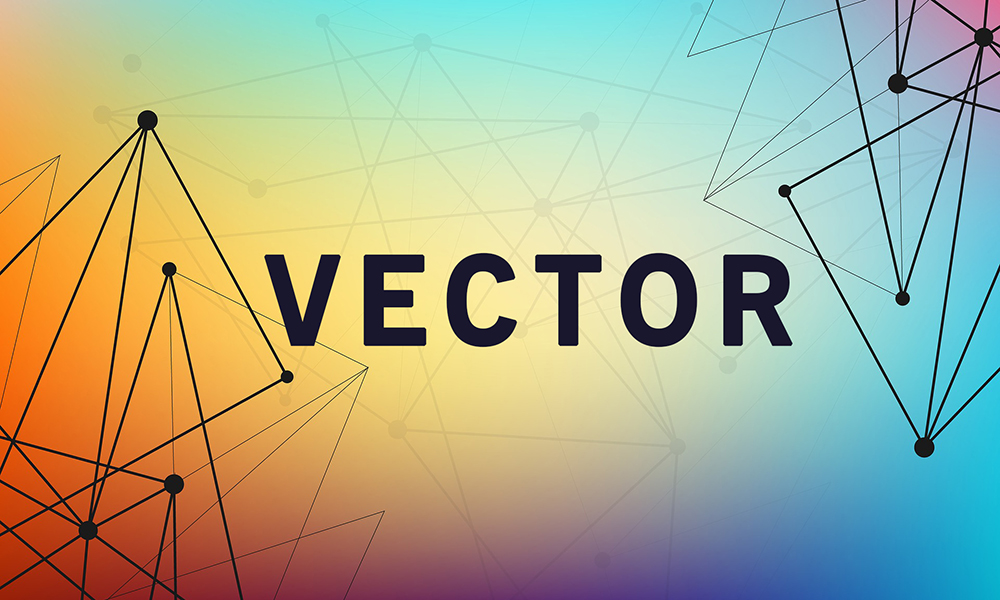The basic determinant of the vector concept is related to the field of mathematics, originally to the so-called linear algebra, and its role, in this case, is related to the differentiation of certain sizes, which certainly occur in nature and which certainly have course, direction, and intensity. Namely, in this area, there are two basic parameters on the basis of which one vector is determined, provided that these are certainly the sizes related primarily to the field of geometry.

However, on this occasion, we are interested in what is meant by the term vector in the field of computing and informatics? Namely, this is the so-called vector graphics, which is also known as geometric design, and it is shortened as a vector, so there is definitely no need for a vector in mathematics and a vector in the field of information technology to be considered identical.

Thus, geometric design implies the way in which a particular image is displayed that includes different geometric shapes, and primarily refers to lines and points, then curves and so-called polygons. There is also a so-called raster or bitmap graphics, which is also significantly more complex than the vector one.

Numerous tests and analyzes have determined that shapes that are made in this way can be much easier to remember, since they are made up of some more simple geometric shapes, but one should bear in mind that exactly the vectors are used when the graphics is to be made into two dimensions, provided it is especially interesting to note that most 3D images actually come from two-dimensional vectors. Unlike raster graphics, vectors allow continuous change, so there is a possibility not only to change the resolution, but also to move, fill, or turn a certain image and on that occasion, the quality of the particular image will not be reduced.

It should be noted that, generally, the size of the vector image is smaller than the raster image, and anyone who wants can zoom in a certain vector segment without the fear that the whole picture quality will be reduced or lost. It is also enabled within the vector graphics to make some changes that are much more demanding, also without reducing the quality.

It is also important to emphasize that vectors are most commonly used in a situation where it is necessary to process an image in a simpler way, and for some more serious changes it is certainly advisable to use raster graphics. As relatively often these two terms are mentioned together, one should emphasize that the difference between vector and raster graphics is essential. First of all, the basis on which the vector graphics function is related to the principles of geometry, more precisely for the vectors, and in this connection, it is also worth pointing out that the vector is defined as the size that has its starting point and its end point, as well as the distance, or direction. It is assumed that if it is a curve, then there are those points within the specific vector by which this line is defined, as well as all the angles it creates. With the application of vector graphics, various geometric shapes are created, such as triangles, circles or squares, and many others. Taking into account that this is a type of graphics primarily based on numerous mathematical functions, this does not diminish the quality of a particular image if it is reduced or enlarged infinitely. That is why very often for the vector, that is, vector graphics we use the symbolic name meaning graphics without losses, or the so-called Loss Less graphics. Most often, this kind of photo processing is used if you need to create a logo for a particular company.

By contrast, raster graphics includes the entire network and vertical and horizontal lines, which make up one field within which pixels are located. So, the image that is processed in this format is made up of a specific number of pixels, each of which has a clearly defined color. When the image processed in this way increases, then its quality changes, becoming much worse, as all pixels become visible to the naked eye.